Examples

Chapter 11 Class 11 - Intro to Three Dimensional Geometry
Serial order wise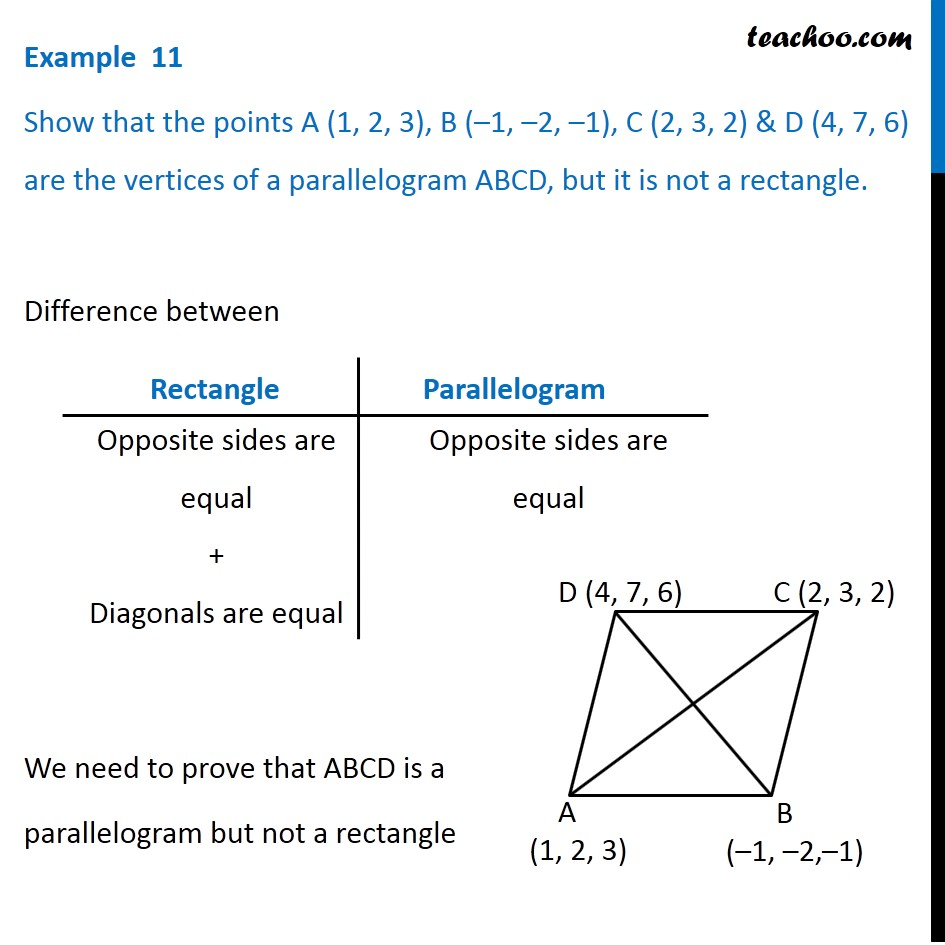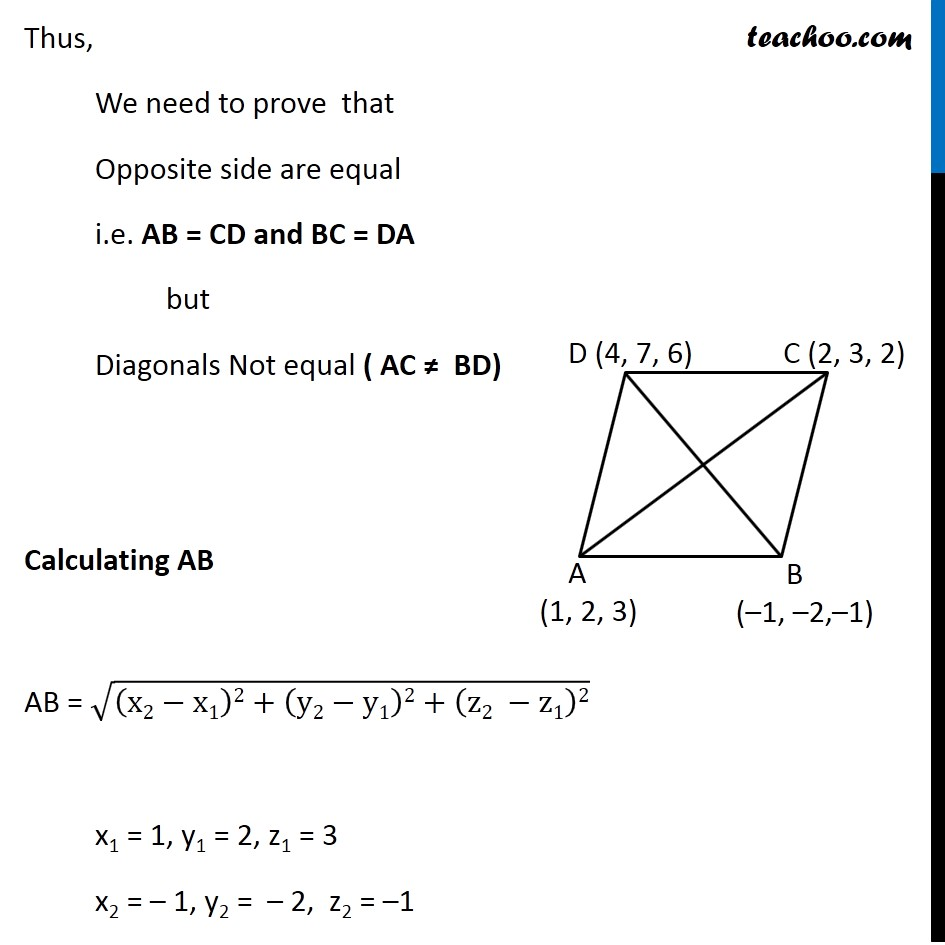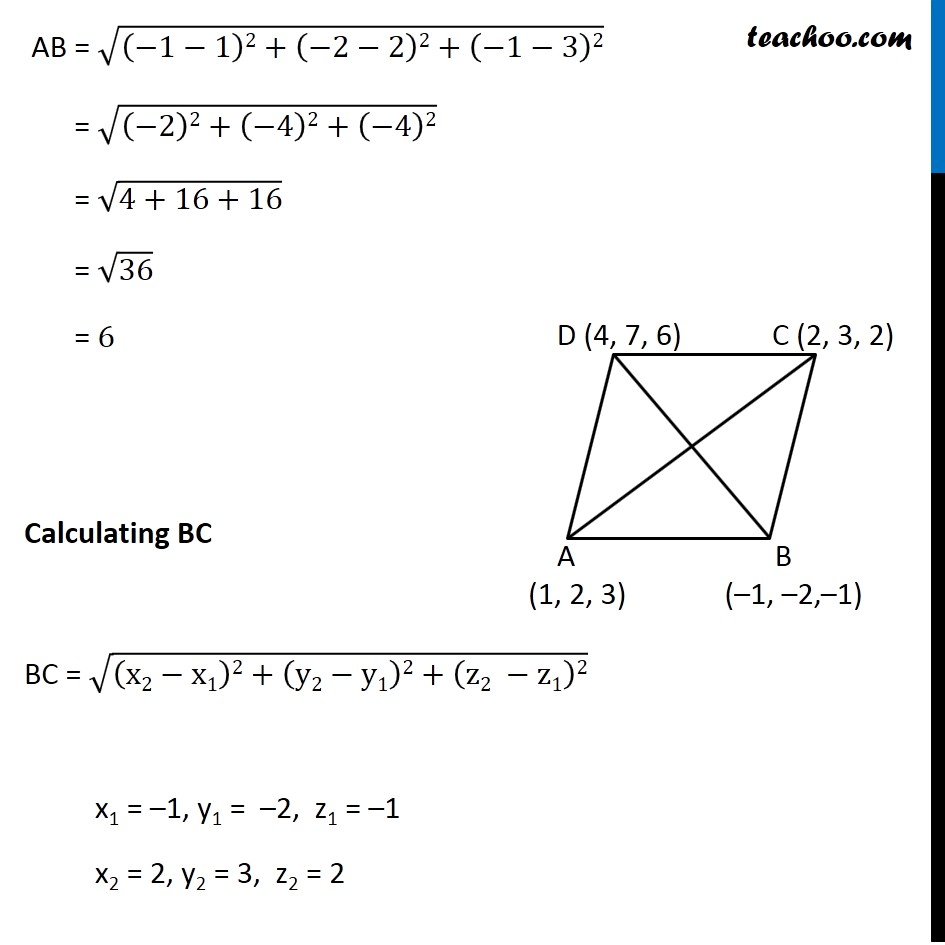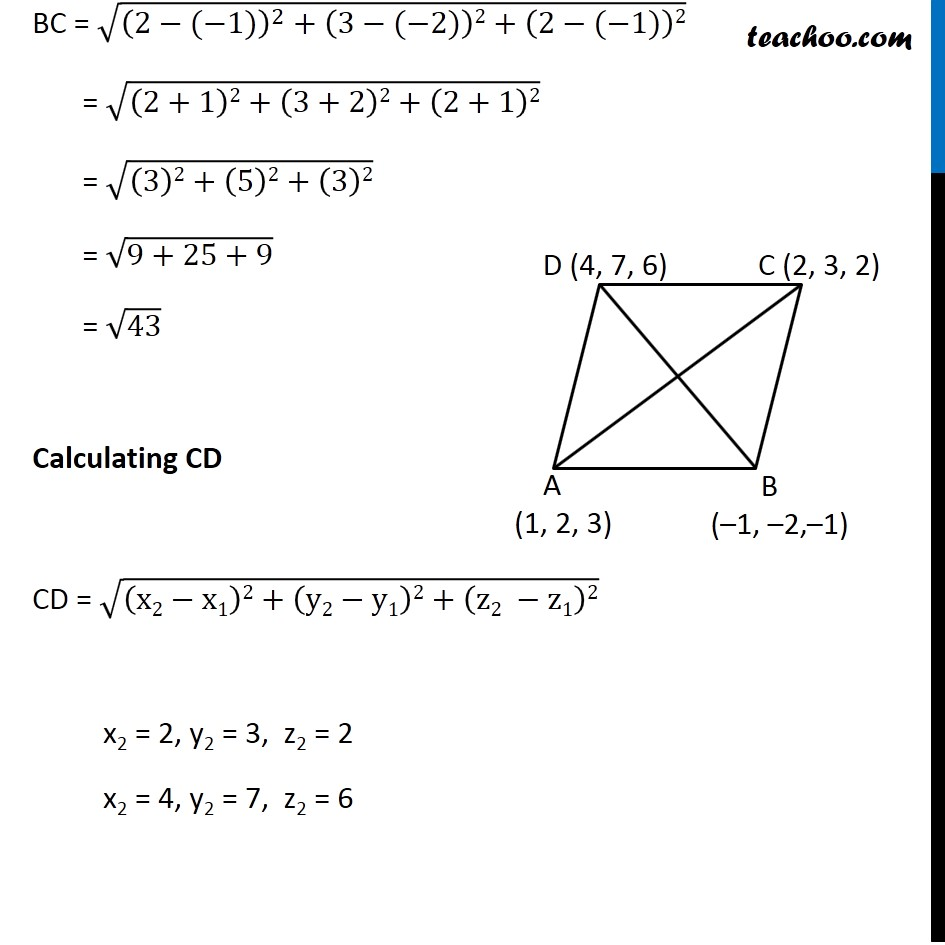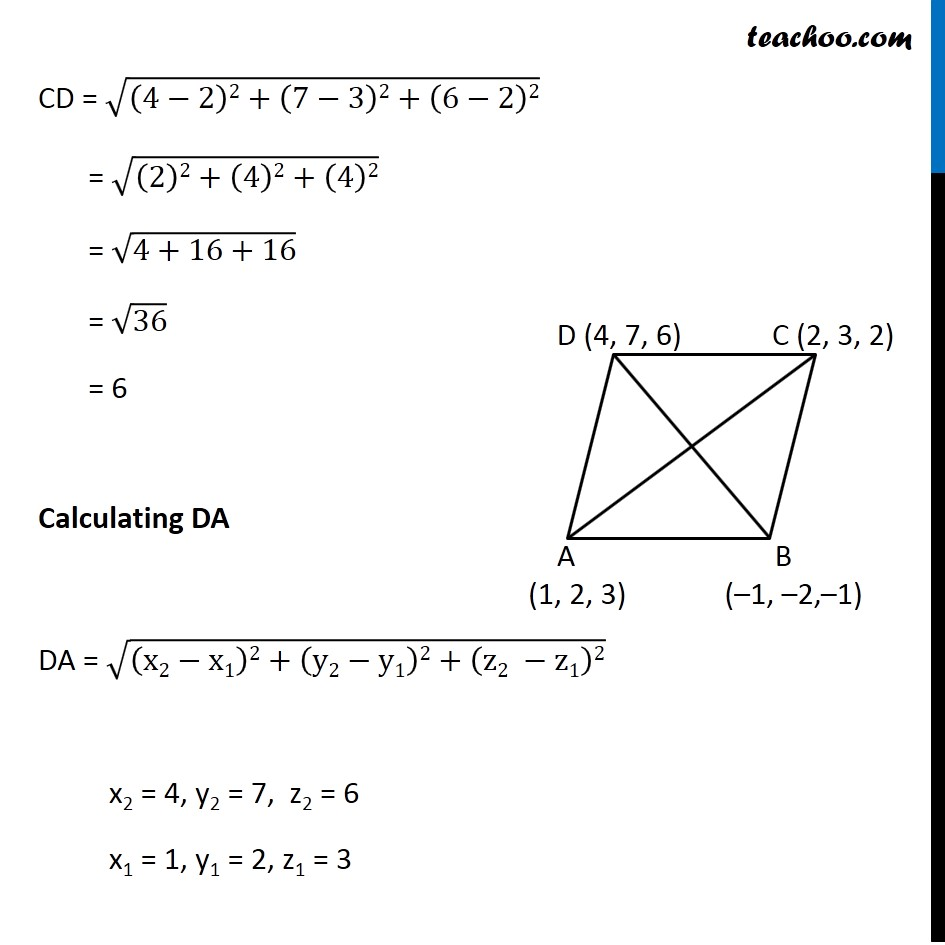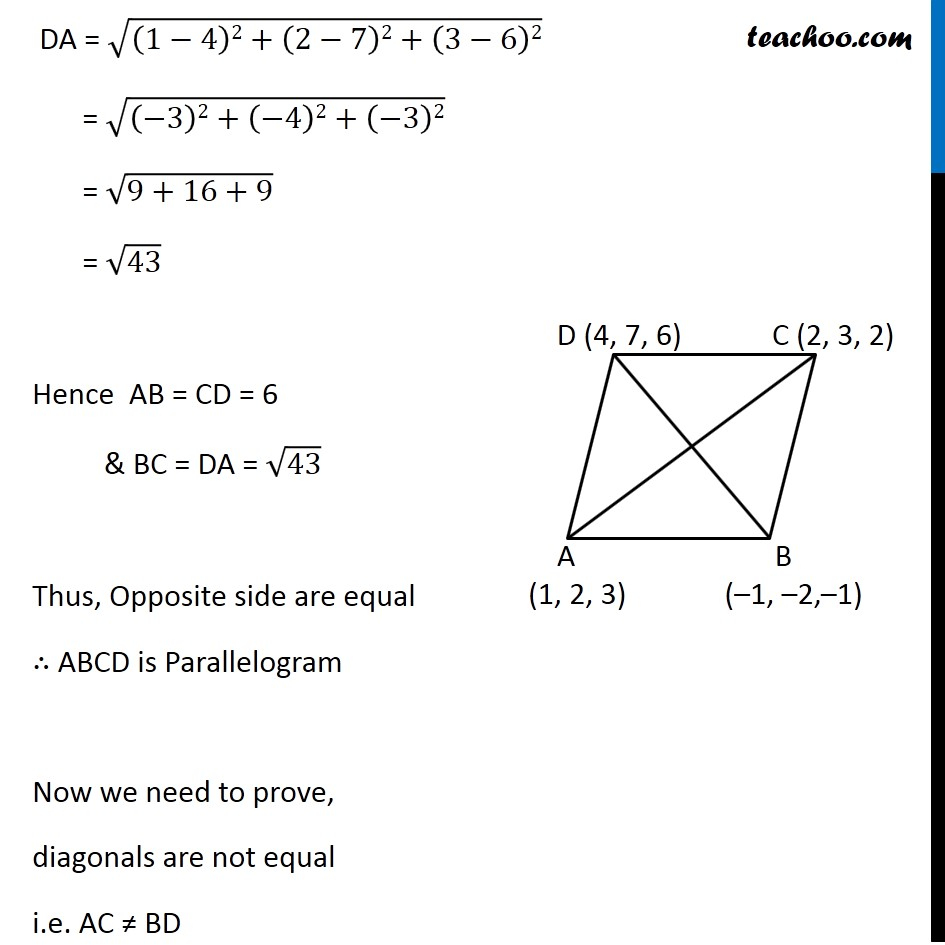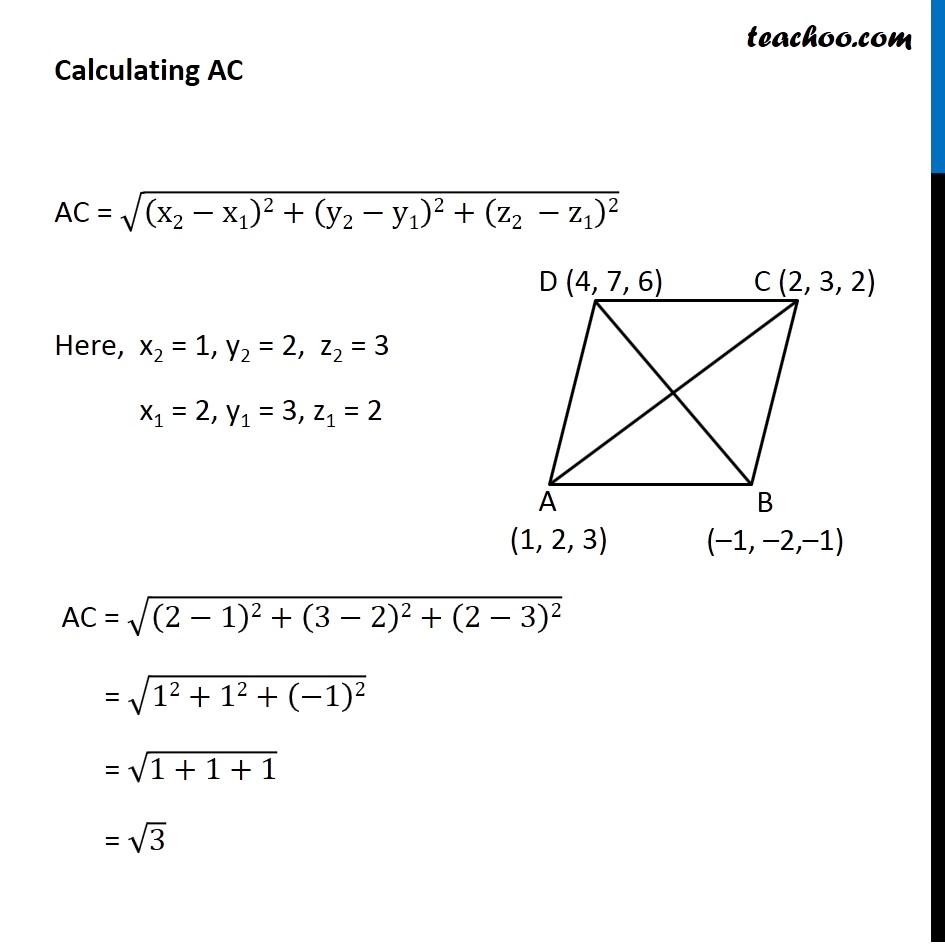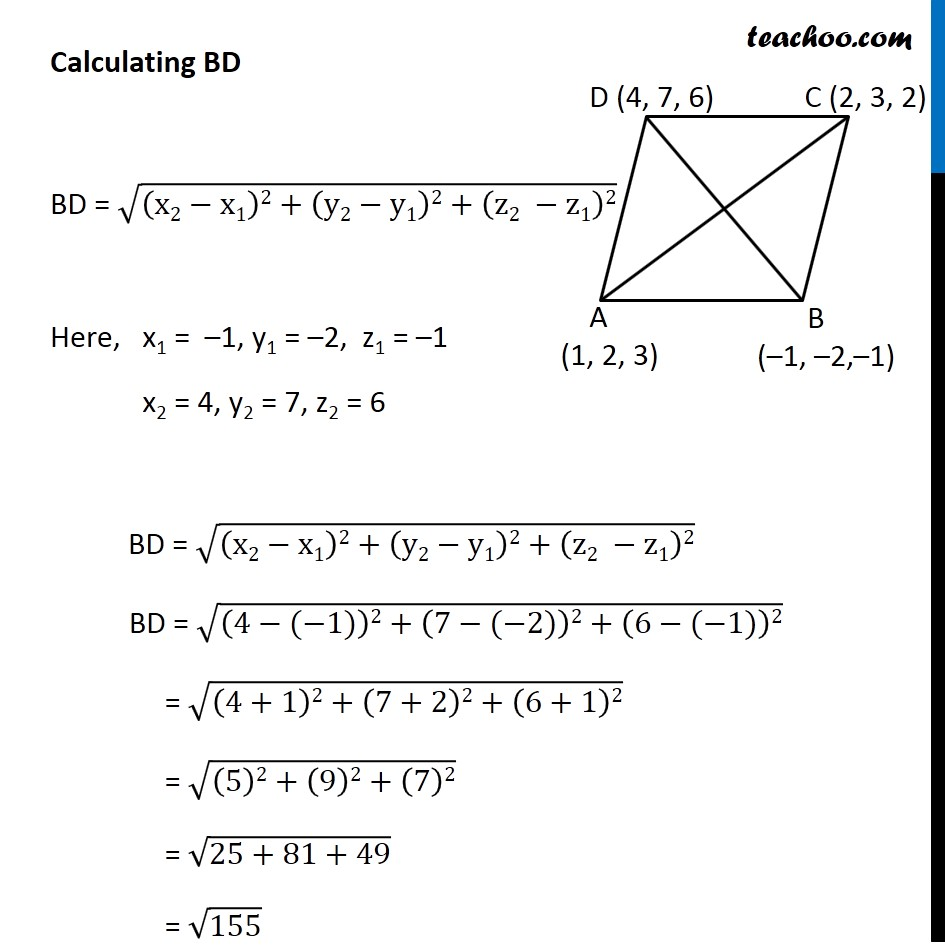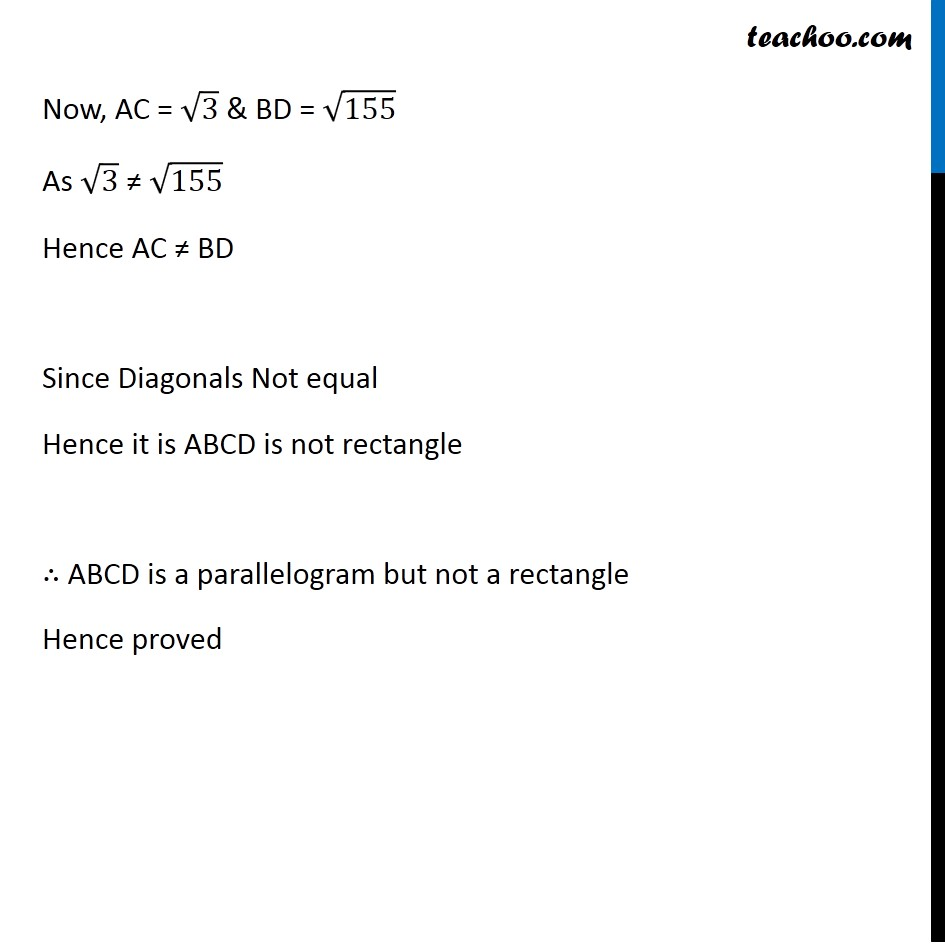Learn in your speed, with individual attention - Teachoo Maths 1-on-1 Class

### Transcript

Example 11 Show that the points A (1, 2, 3), B (–1, –2, –1), C (2, 3, 2) & D (4, 7, 6) are the vertices of a parallelogram ABCD, but it is not a rectangle. Difference between We need to prove that ABCD is a parallelogram but not a rectangle Opposite sides are equal + Diagonals are equal Opposite sides are equal Thus, We need to prove that Opposite side are equal i.e. AB = CD and BC = DA but Diagonals Not equal ( AC ≠ BD) Calculating AB AB = √((x2−x1)2+(y2−y1)2+(z2 −z1)2) x1 = 1, y1 = 2, z1 = 3 x2 = – 1, y2 = – 2, z2 = –1 AB = √((−1−1)2+(−2−2)2+(−1−3)2) = √((−2)2+(−4)2+(−4)2) = √(4+16+16) = √36 = 6 Calculating BC BC = √((x2−x1)2+(y2−y1)2+(z2 −z1)2) x1 = –1, y1 = –2, z1 = –1 x2 = 2, y2 = 3, z2 = 2 BC = √((2−(−1))^2+(3−(−2))2+(2−(−1))2) = √((2+1)2+(3+2)2+(2+1)2) = √((3)2+(5)2+(3)2) = √(9+25+9) = √43 Calculating CD CD = √((x2−x1)2+(y2−y1)2+(z2 −z1)2) x2 = 2, y2 = 3, z2 = 2 x2 = 4, y2 = 7, z2 = 6 CD = √((4−2)2+(7−3)2+(6−2)2) = √((2)2+(4)2+(4)2) = √(4+16+16) = √36 = 6 Calculating DA DA = √((x2−x1)2+(y2−y1)2+(z2 −z1)2) x2 = 4, y2 = 7, z2 = 6 x1 = 1, y1 = 2, z1 = 3 DA = √((1−4)2+(2−7)2+(3−6)2) = √((−3)2+(−4)2+(−3)2) = √(9+16+9) = √43 Hence AB = CD = 6 & BC = DA = √43 Thus, Opposite side are equal ∴ ABCD is Parallelogram Now we need to prove, diagonals are not equal i.e. AC ≠ BD Calculating AC AC = √((x2−x1)2+(y2−y1)2+(z2 −z1)2) Here, x2 = 1, y2 = 2, z2 = 3 x1 = 2, y1 = 3, z1 = 2 AC = √((2−1)2+(3−2)2+(2−3)2) = √(12+12+(−1)2) = √(1+1+1) = √3 Calculating BD BD = √((x2−x1)2+(y2−y1)2+(z2 −z1)2) Here, x1 = –1, y1 = –2, z1 = –1 x2 = 4, y2 = 7, z2 = 6 BD = √((x2−x1)2+(y2−y1)2+(z2 −z1)2) BD = √((4−(−1))2+(7−(−2))2+(6−(−1))2) = √((4+1)2+(7+2)2+(6+1)2) = √((5)2+(9)2+(7)2) = √(25+81+49) = √155 Now, AC = √3 & BD = √155 As √3 ≠ √155 Hence AC ≠ BD Since Diagonals Not equal Hence it is ABCD is not rectangle ∴ ABCD is a parallelogram but not a rectangle Hence proved